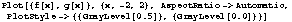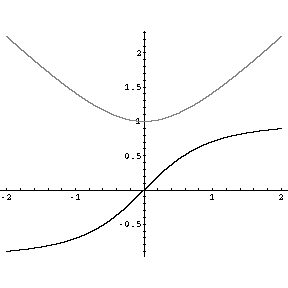A Sample Syllabus

This file has been created by Mathematica 3.0 and saved in the html format (use Notebook->Save As Special->HTML menu). You may consider this method of posting files that contain some mathematical equations or figures. Note that this is not a live  Mathematica file (i.e. you cannot execute commands directly from this file), but in creating the file, power of Mathematica was at the author's disposal.

This simple example computes the derivative of f(x)=, and then plots both the function and its derivative on the same coordinate system.

We begin by defining the function.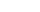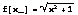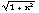Now we compute the derivative, naming it g(x).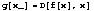Finally, we will plot the two functions. The domain for the plot is chosen arbitrarily as the interval (-2,2) (hence {x,-2,2}), the scale on both axes is set the same (AspectRatio->Automatic), the function f(x) is displayed in gray, while the derivative is in black (PlotStyle->{{GrayLevel[0.5]},{GrayLevel[0.0]}}).